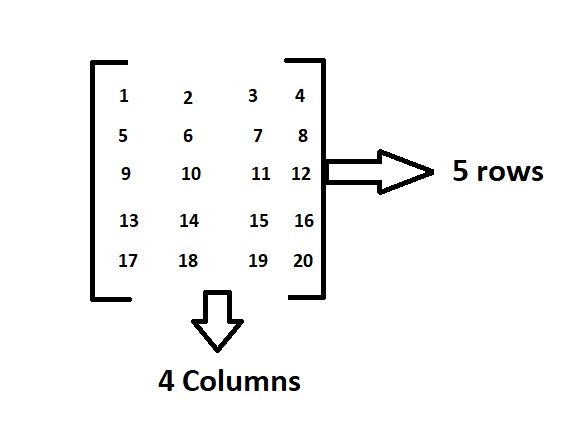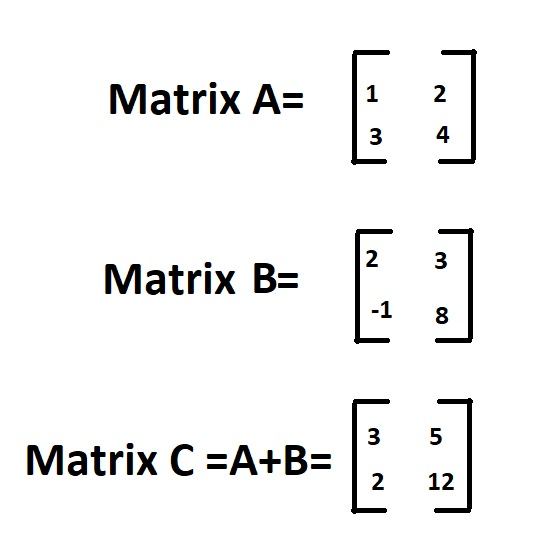# Program for addition of two matrices in Python & C++ Programming

In the previous article, we have discussed about C++11 Multithreading – Part 8: std::future , std::promise and Returning values from Thread. Let us learn Program for addition of two matrices in C++ Program and Python.

What is a matrix:

A matrix is a rectangular sequence of numbers divided into columns and rows. A matrix element or entry is a number that appears in a matrix.

Example:Above is the matrix which contains 5 rows and 4 columns and having elements from 1 to 20.

In this order, the dimensions of a matrix indicate the number of rows and columns.

Here as there are 5 rows and 4 columns it is called as 5*4 matrix.

Given two matrices of same order(dimensions) then we can easily add the two matrices by doing the sum of corresponding elements in both the matrices.

Example:Here Matrix C is matrix addition of the matrices A and B.

Input:

Matrix 1  =  [  11  -2    0  ]
[   4    8    6  ]
Matrix 2 =   [   5    1   -5  ]
[   2    3    0 ]

Output:

Printing the sum of matrices :
16 -1 -5
6 11 6

Drive into Python Programming Examples and explore more instances related to python concepts so that you can become proficient in generating programs in Python Programming Language.

### Method #1:Using nested Loops in Python

A matrix can be implemented as a nested list in Python (list inside a list). Each variable can be thought of as a row in the matrix.

We used nesting loops in this program to iterate through and row and column. In the two matrices we will add the appropriate elements and store them in the result at each level.

Here we give the matrix input as static.

Below is the  implementation:

# given matrix A
A = [[11, -2, 0],
[4, 8, 6]]
# given matrix B
B = [[5, 1, -5],
[2, 3, 0]]
# Initialize the sum of matrices elements to 0
matrixSum = [[0, 0, 0],
[0, 0, 0]]

# Traverse the rows
for rows in range(len(A)):
# Traverse the  columns
for columns in range(len(A)):
matrixSum[rows][columns] = A[rows][columns] + B[rows][columns]
# printing the sum of matrices
print("Printing the sum of matrices : ")
for rows in matrixSum:
print(*rows)


Output:

Printing the sum of matrices :
16 -1 -5
6 11 6

### Method #2:Using List Comprehension in Python

The program’s output is the same as previously. For iterating through each element in the array, we used the nested list comprehension.

Understanding the list helps us to write concise codes and we need to always try to use them in Python. They’re really beneficial.

Below is the implementation:

# given matrix A
A = [[11, -2, 0],
[4, 8, 6]]
# given matrix B
B = [[5, 1, -5],
[2, 3, 0]]
# using list comprehension
matrixSum = [[A[i][j] + B[i][j]
for j in range(len(A))] for i in range(len(A))]
# printing the sum of matrices
print("Printing the sum of matrices : ")
for rows in matrixSum:
print(*rows)


Output:

Printing the sum of matrices :
16 -1 -5
6 11 6

### Method #3:Using nested loops in C++

We used nesting loops in this program to iterate through and row and column. In the two matrices we will add the appropriate elements and store them in the result at each level.

Let us take dynamic input in this case.

Below is the implementation:

#include <iostream>
using namespace std;

int main()
{
int rows, columns, A, B, sumMatrix, i, j;

cout << "Enter the number of rows of the matrix "<<endl;
cin >> rows;

cout << "Enter the number of columns of the matrix"<<endl;
cin >> columns;

cout << "Enter the elements of first matrix " << endl;

// Initializing matrix A with the user defined values
for(i = 0; i < rows; ++i)
for(j = 0; j < columns; ++j)
{
cout << "Enter element A" << i + 1 << j + 1 << " = ";
cin >> A[i][j];
}

// Initializing matrix B with the user defined values
cout << endl << "Enter elements of 2nd matrix: " << endl;
for(i = 0; i < rows; ++i)
for(j = 0; j < columns; ++j)
{
cout << "Enter element B" << i + 1 << j + 1 << " = ";
cin >> B[i][j];
}

// Performing matrix addition by doing sum of given two matrices A and B
for(i = 0; i < rows; ++i)
for(j = 0; j < columns; ++j)
sumMatrix[i][j] = A[i][j] + B[i][j];
//printing matrix A
cout << endl << " printing the matrix A" << endl;
for (i = 0; i < rows; ++i) {
for (j = 0; j < columns; ++j) {
cout << A[i][j] << "  ";
}
cout << endl;
}
//printing matrix B
cout << endl << " printing the matrix B" << endl;
for (i = 0; i < rows; ++i) {
for (j = 0; j < columns; ++j) {
cout << B[i][j] << "  ";
}
cout << endl;
}

//printing the sum of matrices
cout << endl << " printing the sum of matrices A and B" << endl;

for (i = 0; i < rows; ++i) {
for (j = 0; j < columns; ++j) {
cout << sumMatrix[i][j] << "  ";
}
cout << endl;
}

return 0;
}

Output:

Enter the number of rows of the matrix
3
Enter the number of columns of the matrix
3
Enter the elements of first matrix
Enter element A11 = 1
Enter element A12 = 2
Enter element A13 = 3
Enter element A21 = 4
Enter element A22 = 5
Enter element A23 = 6
Enter element A31 = 7
Enter element A32 = 8
Enter element A33 = 9

Enter elements of 2nd matrix:
Enter element B11 = -3
Enter element B12 = 2
Enter element B13 = 5
Enter element B21 = 7
Enter element B22 = 1
Enter element B23 = 0
Enter element B31 = 3
Enter element B32 = 4
Enter element B33 = 6

printing the matrix A
1 2 3
4 5 6
7 8 9

printing the matrix B
-3 2 5
7 1 0
3 4 6

printing the sum of matrices A and B
-2 4 8
11 6 6
10 12 15

Related Programs: